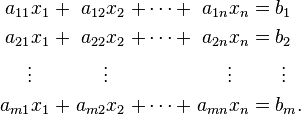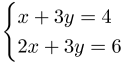## System Solver

A system of linear equations is a collection of linear equations involving the same set of variables. A general system of m linear equations with n unknowns can be written as:Here, x1, x2, …, xn are the unknowns, a11, a12, …, amn are the coefficients of the system, and b1, b2, …, bm are the constant terms. (Source: Wikipedia)

Write a program that solves a system of linear equations with a maximum of 100 equations and variables.

### Input Format

Line 1 of the input contains integers n and m (1 ≤ n, m ≤ 100), indicating the number of variables to solve for and the number of equations in the system.
The next m lines will each contain n+1 integers, where the first n integers are the coefficients of the equation and the last integer is the constant term.
Every number in the input is guaranteed to fit in a 32-bit signed integer.

### Output Format

If the system can be solved, output n lines, the values of the unknowns x1, x2, …, xn, accurate within ±10-5.
If there are no solutions to the system, or if there are infinite solutions to the system, output "NO UNIQUE SOLUTION".

### Sample Input 1

2 2
1 3 4
2 3 6


### Sample Output 1

2
0.66667


### Explanation

This asks for the solution(s) for x in the system:$\displaystyle \begin{cases} x + 3y = 4 \\ 2x + 3y = 6 \\ \end{cases}$
Solving for x in the first equation gives x = 4 - 3y. Substituting this into the 2nd equation and simplifying yields 3y = 2.
Solving for y yields y = 23. Substituting y back into the first equation and solving for x yields x = 2.
Therefore the solution set is the single point (x, y) = (2, 23).

### Sample Input 2

2 3
6 2 2
12 4 8
6 2 4


### Sample Output 2

NO UNIQUE SOLUTION


### Explanation

All of the lines are parallel. Therefore, the system of equations cannot be solved.

Point Value: 20 (partial)
Time Limit: 2.00s
Memory Limit: 16M